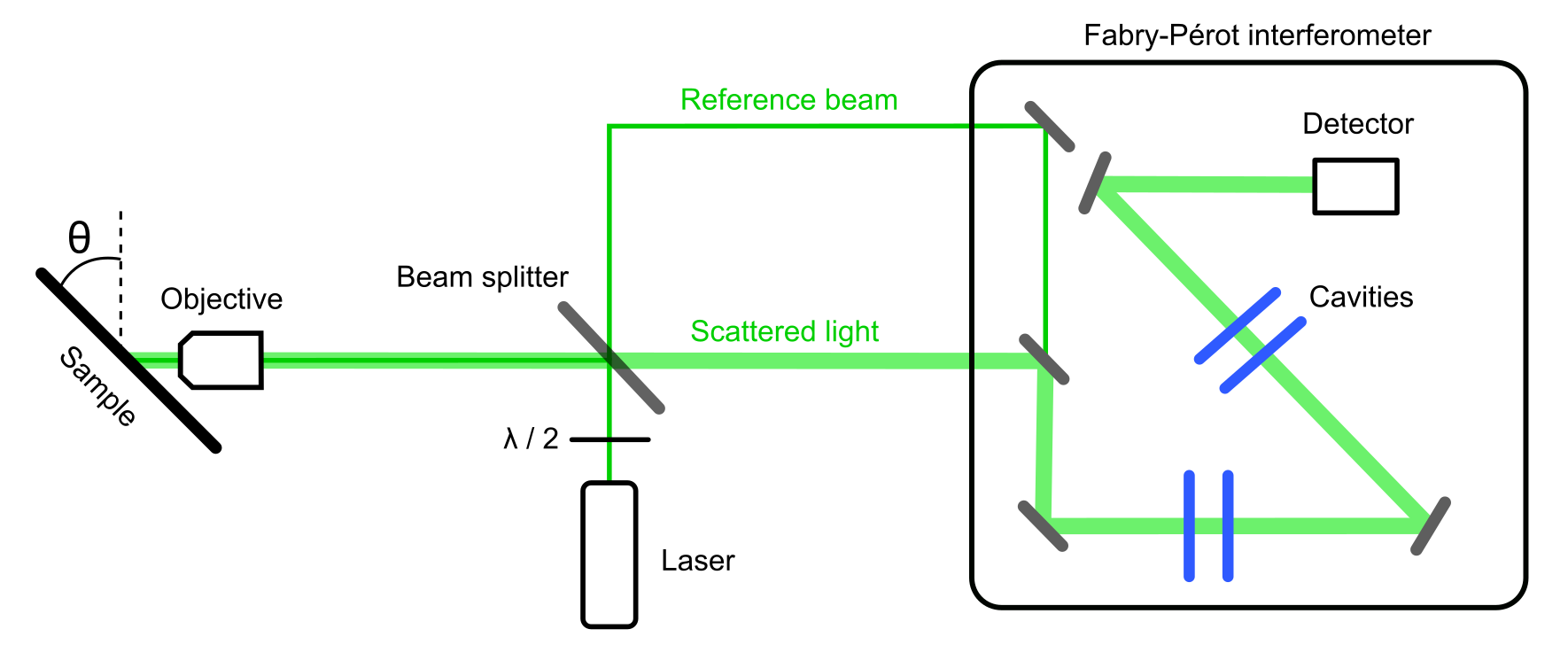# Brillouin light scattering spectroscopy

Brillouin light scattering (BLS) spectroscopy is an experimental method to measure the frequency spectra of elastic modes for a given wavevector. This method derives its name from inelastic Brillouin scattering of light on the acoustic phonons of the scattering material. Such scattering changes the energy of the scattered light, so that the detected wavelength is shifted positively (Stokes shift) or negatively (anti-Stokes shift) as compared to the incident light. To measure the tiny shift in the wavelength, the BLS method used Fabry–Pérot interferometer. In the interferometer, the scattered light is passing through a pair of cavities, each consisting of two parallel mirrors. Light wave can pass through a cavity only when the wave is in a resonance with the cavity. Thus, the interferometer can scan a range of wavelengths.The wavevector, for which the phonon spectrum is measured, can be chosen by changing the angle between the incident beam and the surface. Specifically, the wavevector $q$ is given by

$q = 4 \pi sin(\theta)/\lambda$,

where $\theta$ is the incidence angle and $\lambda$ is the wavelength of incident light.

• F. Kargar and A. Balandin “Advances in Brillouin–Mandelstam light-scattering spectroscopy” Nature Photonics, 15 720-731 (2021)